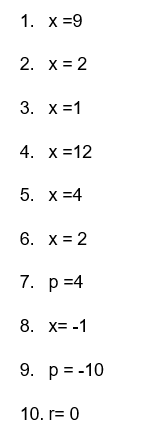# Linear Equations in One Variable Class 8 Worksheet (with answer key + PDF)

A mathematical statement known as an equation contains two expressions separated by an equal sign. The value of the expression to the left of the equal sign is the same as the expression to the right. When a linear equation is graphed, it always produces a straight line because each term in the equation has an exponent of 1. There are both linear equations with one variable and those with two.

What is the “Linear Equations in One Variable Class 8 Worksheet with answers (PDF)”?

This worksheet will contain Linear Equations in One Variable. In a linear equation, there have two variables but in this section, we will discuss is Linear equation in One variable.

## What is Linear Equation in one variable?

The definition of a linear equation in one variable is an equation in which every term’s highest degree is one, the equation has only one possible solution, and there is only one variable present.

This worksheet will help you solve a linear equation that includes a set of simple methods.

Instructions on how to use the “Linear Equations in One Variable Class 8 Worksheet with answers (PDF).”

Students should study the concept and the examples given, then attempt to finish the exercises below, in order to comprehend the Linear Equation completely.

## Conclusion

The fundamental equation used to represent and solve for an unknown quantity is a linear equation in one variable. It is always a straight line, and it is easily represented graphically. A mathematical statement is simply represented by a linear equation. Unknown quantities can be represented by any variable or symbol, but typically, the unknown quantity in a linear equation with one variable is represented by the variable “x.”

If you have any inquiries or feedback, please let us know.

## Linear Equations in One Variable Class 8 Worksheet with answer key + PDF)

A linear Equation is an equation of a straight line. The highest degree of power is 1. A linear equation in one variable is an equation that can be written in the form ax+b=0 and when this equation is graphed, it always results in a straight line.

The graph of a linear equation with one variable, x, forms a straight line that is vertical and parallel to the y-axis.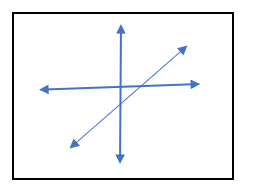A linear equation with one variable has the general form ax + b = 0.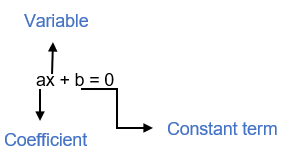For example, 2x+7=19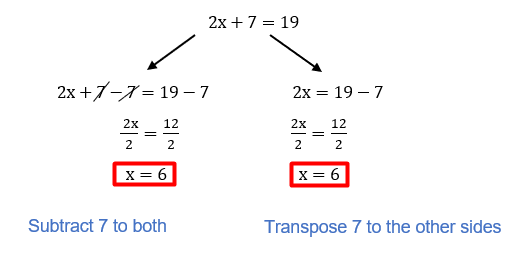1. Solving Linear Equations in one variable.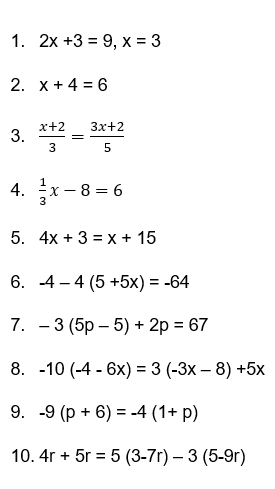1. Reflection

What do you think about solving the Linear Equations in one variable? Did you find it difficult to follow the pattern in each step?